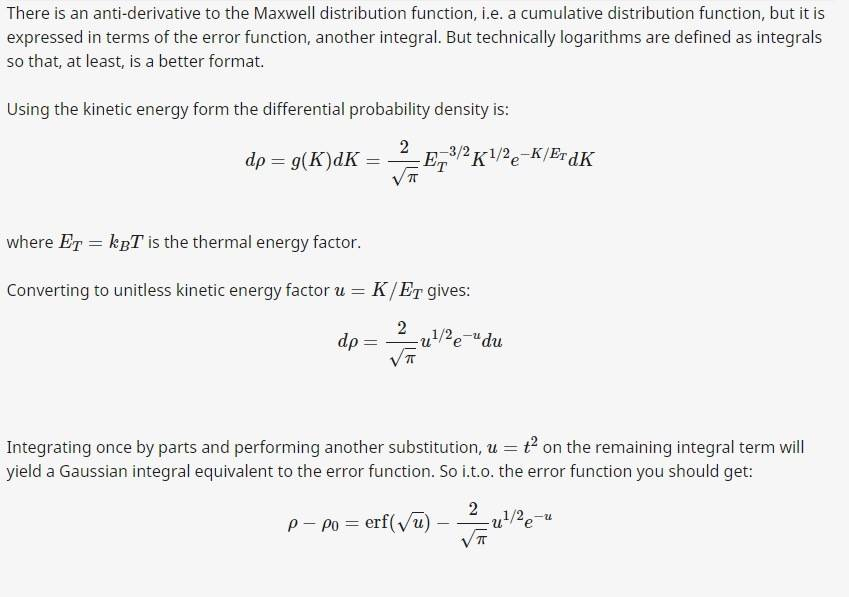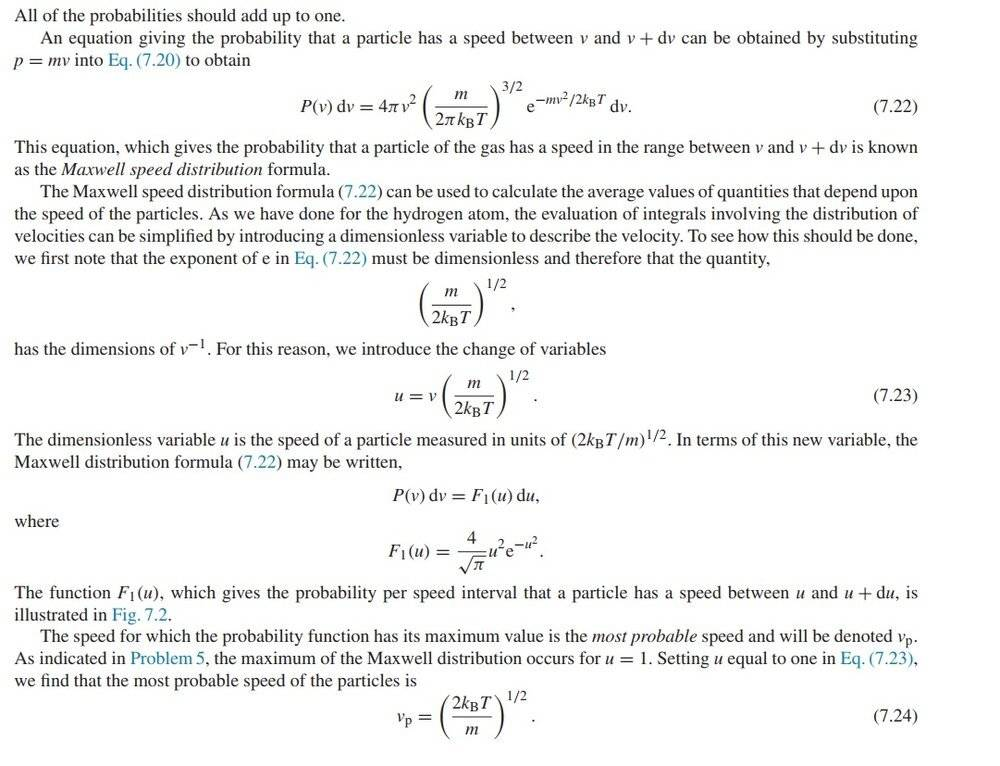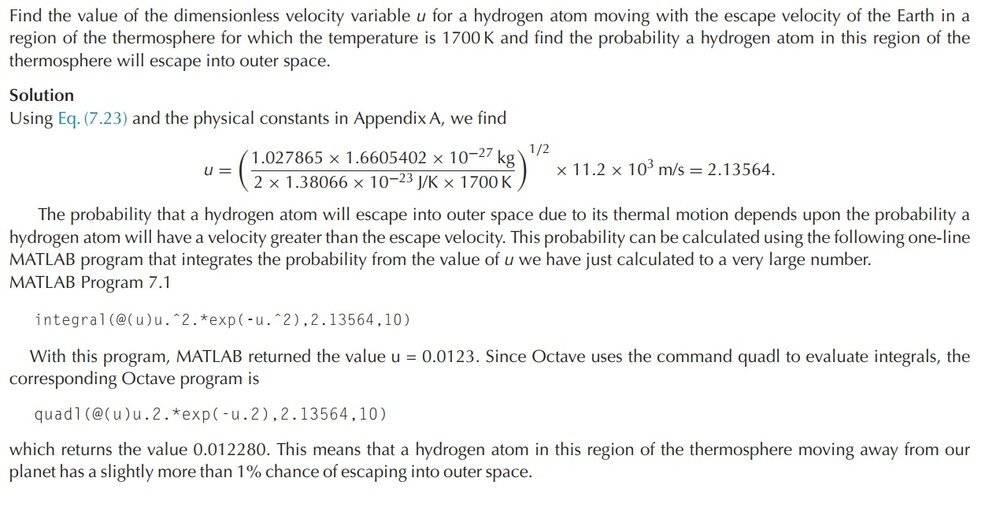# Integration of the Maxwell Speed Distribution

• A
• TeslaPow

#### TeslaPow

Need some help on how to solve the integration formula for Maxwell speed distribution, here is the procedure on
how to solve for the kinetic energy:Not familiar with the error function yet, but the result for the kinetic energy integration is:

https://www.wolframalpha.com/input/?i=d/du(erf(sqrt(u))-2/(sqrt(pi))*sqrt(u)*e^(-u))

How to go on about solving for the speed distribution is the topic for this problem.

http://integral-table.com/Last edited:

What do you mean by "solving for the speed distribution"? That's given in equation 7.22.

Trying to use the dimensionless variable u in the same manner as in the calculation for integrating between two energy levels as for the distribution of speeds. If I pick a random gas with different velocities and integrate between these, the numbers don't turn up the same.

For energy integral you can use u = K/E, and integrate between energy levels. As it is an probability distribution, all of the probabilities should add up as one in the definite integral. The answer will come out as percentage.

https://www.wolframalpha.com/input/?i=d/du(erf(sqrt(u))-2/(sqrt(pi))*sqrt(u)*e^(-u))

The definite integral for the distribution of speeds turns up as 0.443

https://www.wolframalpha.com/input/?i=d/du(-1/2+e^(-u^2)+u+++1/4+sqrt(π)+erf(u))

Last edited:
In response to your last line, don't you see in your first post that there is a factor of ## \frac{4}{\sqrt{\pi}} ## in the speed distribution function? It thereby is properly normalized.

•etotheipi
In response to your last line, don't you see in your first post that there is a factor of ## \frac{4}{\sqrt{\pi}} ## in the speed distribution function? It thereby is properly normalized.

Here's an example, why is it excluded here ?Last edited:
Without studying it in great detail, I think they goofed. They need to include the normalization factor.

•etotheipi
Without studying it in great detail, I think they goofed. They need to include the normalization factor.

In the text, for equation 7.23, it says that the normalization factor is taken into account. v^(-1)

In the text, for equation 7.23, it says that the normalization factor is taken into account. v^(-1)
In the solution presented, they integrate ##u^2 \exp(-u^2)##, then say that the result is the probability. This is incorrect, as they forgot the normalization factor. The correct probability is the result they obtained multiplied by ##4/\sqrt{\pi}##, which is 0.0277.

•Look at your "link" in at the bottom of post 4. That one has it right for the speed distribution, where at the bottom of the "link" they compute the integral from ## 0 ## to ## + \infty ##, and the inverse of that is the normalization factor.
If you look at your first post between 7.23 and 7.24, they also have the correct expression for ## F_1(u) ##.

Last edited:
Dr. Claude and Charles have both pointed out that the book made a mistake by omitting the normalization constant, but for whatever reason, you don't seem to accept that as the reason. It may help to work the problem out by hand. Just integrate the expression given for ##F_1(u)## from 0 to ##\infty##, and you'll see the result is equal to 1. You can do it by integrating by parts. Or can you explain why you just keep repeating your original question, which already has been answered? In other words, what's your real question?

Also, if you take the expression for ##d\rho## and actually do the change of variables mentioned in the text, you'll get the expression given for ##F_1##.

••